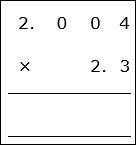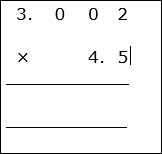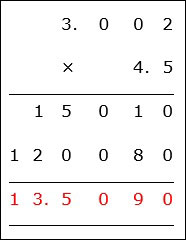# Decimal Multiplication: Problem Type 2

Multiplication of decimal numbers is similar to whole number multiplication.

Rules for Decimal Multiplication

• First we multiply the decimal numbers as if they were whole numbers by ignoring the decimal points.

• Here the decimal points are not lined up vertically as in addition and subtraction. Here the digits are lined up to the right.

• The number of digits after the decimal point in both the numbers are counted and added.

• In the final product, a decimal point is placed before that many digits from the right.

Multiply 2.004 × 2.3

### Solution

Step 1:

2.004 × 2.3

Here the digits are lined up to the rightWe now multiply the numbers by ignoring the decimal points and assuming as if they were whole numbers.

Step 2:

2.004 has three decimal places. 2.3 has one decimal place. So the product of these numbers has four decimal places.Multiply 3.002 × 4.5

### Solution

Step 1:

3.002 × 4.5

Here the digits are lined up to the rightWe now multiply the numbers by ignoring the decimal points and assuming as if they were whole numbers.

Step 2:

3.002 has three decimal places. 4.5 has one decimal place. So the product of these numbers has four decimal places.